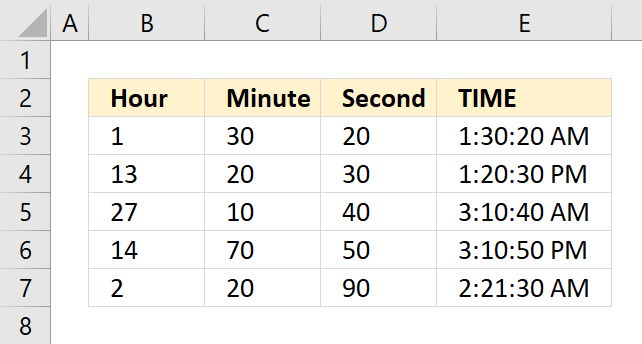Author: Oscar Cronquist Article last updated on June 17, 2018The TIME function returns a decimal value between 0 (zero) representing 12:00:00 AM and 0.99988426 representing 11:59:59 P.M.

Formula in cell D3:

=TIME(B3, C3, D3)

### Excel Function Syntax

TIME(hour, minute, second)

### Arguments

 hour Required. A number between 0 and 32767 that represents the hour. minute Required. A number between 0 and 32767 that represents the minute. second Required. A number between 0 and 32767 that represents the second.

### Comment

An hour value greater than 23 will be divided by 24 and the remaining hours will be returned by the function. See row 5 in the picture above.

A minute value greater than 59 will be divided by 60 and the remaining minutes will be returned by the function. See row 6 in the picture above.

A "second" value greater than 59 will be divided by 60 and the remaining seconds will be returned by the function. See row 7 in the picture above.